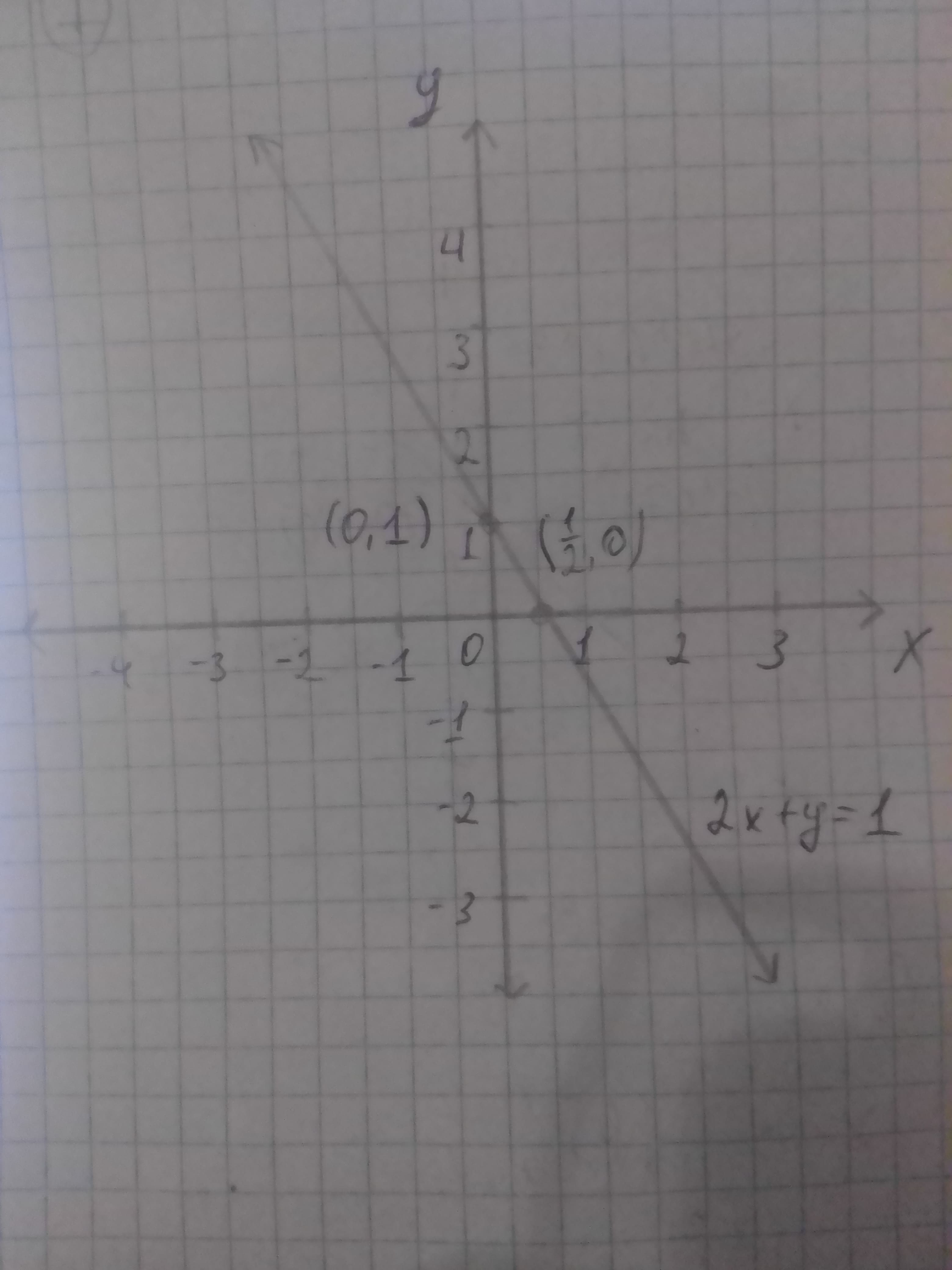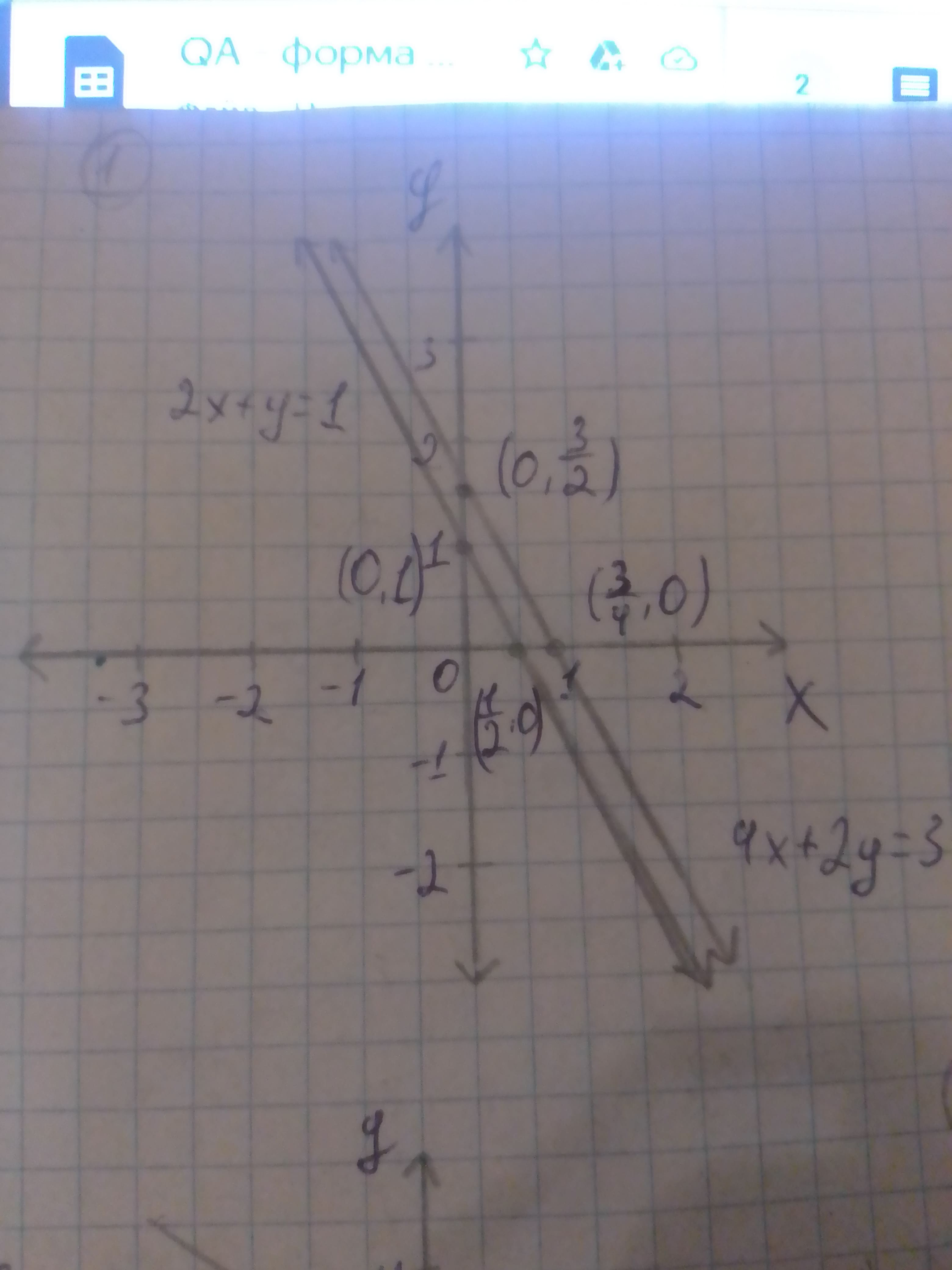Reggie

2021-02-27

The system of equation $\left\{\begin{array}{l}2x+y=1\\ 4x+2y=3\end{array}$ by graphing method and if the system has no solution then the solution is inconsistent.
Given: The linear equations is $\left\{\begin{array}{l}2x+y=1\\ 4x+2y=3\end{array}$lobeflepnoumni

Calculation:
The first equation is given below.
$2x+y=1\dots \left(1\right)$
The second equation is given below
$4x+2y=3\dots \left(2\right)$
Step 1:
Write the equation (1) in slope-intercept standard form as follows:
$2x+y=1$
$y=1-2x$
Compare the equation $y=-2x+1$ with the slope-intercept form $y=mx+c$.
$m=-2$
$c=1$
Therefore, the value of slope m is -2 and c is 1.
Thus, the value of y-intercept (0, c) is (0, 1).
Subtitute 0 for y in equation $y=-2x+1$ to obtain the value of x-intercept.
$0=-2x+1$
$-1=-2x$
$x=\frac{1}{2}$
Therefore, the x-intercept is $\left(\frac{1}{2},0\right).$
Join the points (0, 1) and $\left(\frac{1}{2},0\right)$ to make a line on the graph as shown below in Figure 1:Step 2:
Write the equation (2) in slope-intercept standard form as follows:
$4x+2y=3$
$2y=-4x+3$
$y=-\frac{-4}{2}x+\frac{3}{2}$
$y=-2x+\frac{3}{2}$
Compare the equation $y=-2x+\frac{3}{2}$ with the slope-intercept form $y=mx+c$
. $m=-2$
$c=\frac{3}{2}$
Therefore, the value of slope m is -2 and c is $\frac{3}{2}$
Thus, the value of y-intercept (0, c) is $\le \left(\frac{{x}^{2}}{{y}^{3}}\right)$
Substitute 0 for y in equation $y=-2x+\frac{3}{2}$ to obtain the value of x-intercept.
$0=-2x+\frac{3}{2}$
$-\frac{3}{2}=-2x$
$x=\frac{3}{4}$
Thus, the x-intercept is $\left(0,\frac{3}{4}\right)$
Join the points $\left(0,\frac{3}{2}\right)\phantom{\rule{1em}{0ex}}\text{and}\phantom{\rule{1em}{0ex}}\left(\frac{3}{4},0\right)$ to make a line on the same graph of Figure 1 then the updated graph is shown below in Figure 2.Step 3:
From Figure 2, it is observed that there is no point of intersection of lines.
Therefore, the lines never meet and are parallel to each so the system has no solution in the systems of linear equations.
Thus, the system is inconsistent and the system has no solution as shown below in Figure 2.

Do you have a similar question?